Picture by Leone Venter, Unsplash.

The boxplot, or box-and-whisker diagram, is one of the most useful ways to visualise statistical distributions in data. While they can seem a bit unintuitive when you first look at them, boxplots actually tell you a great deal about the underlying data and are fairly easy to interpret when you know how.

### How to interpret a boxplot

A boxplot divides data into quartiles, with the box representing the interquartile range. The mid-line of the box (which isn’t always central) represents the median; the top of the box represents the upper quartile (Q3), and the bottom represents the lower quartile (Q1).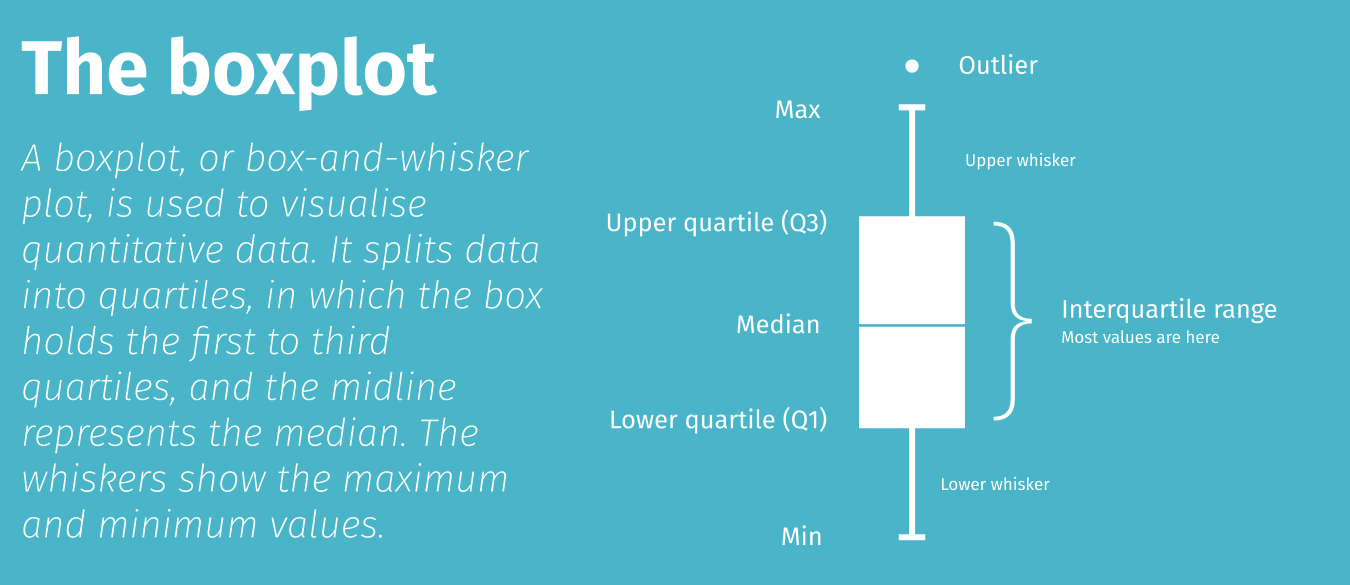The lines at the top and bottom are called whiskers and represent the tail of the data either side of the interquartile range. The ends of the whiskers represent the minimum and maximum values in the data set, although outliers often appear just off the edges.

The boxplot (or boxplots) are shown on an axis to show where the values lie. A short boxplot means the data are concentrated around a smaller range, while a longer boxplot and longer whiskers, indicating a broader range and longer tails.

For this project we’ll be using Pandas for viewing dataframes of raw data and Seaborn for visualising the data in boxplots. Seaborn is a wrapper around Matplotlib and makes it much quicker and easier to create aesthetically pleasing data visualisations. To make these a bit sharper on retina or 4K displays, we will pass in a couple of custom settings to increase the pixel density.

``````import pandas as pd
import seaborn as sns
``````
``````%config InlineBackend.figure_format = 'retina'
sns.set_context('notebook')
``````

Seaborn comes with a couple of datasets which are ideal for plotting data using boxplots. The Iris data set included taxonomic data on the measurements of iris foliage, while the Tips data set includes tips made by restaurant diners. We’ll load each data set into a named dataframe.

``````df_iris = sns.load_dataset('iris')
``````
sepal_length sepal_width petal_length petal_width species
0 5.1 3.5 1.4 0.2 setosa
1 4.9 3.0 1.4 0.2 setosa
2 4.7 3.2 1.3 0.2 setosa
3 4.6 3.1 1.5 0.2 setosa
4 5.0 3.6 1.4 0.2 setosa
``````df_tips = sns.load_dataset('tips')
``````
total_bill tip sex smoker day time size
0 16.99 1.01 Female No Sun Dinner 2
1 10.34 1.66 Male No Sun Dinner 3
2 21.01 3.50 Male No Sun Dinner 3
3 23.68 3.31 Male No Sun Dinner 2
4 24.59 3.61 Female No Sun Dinner 4

### Single boxplot

To create a single boxplot, all you need to do is call the `boxplot()` function and pass in the `y` argument with the Pandas dataframe column you wish to plot. By default, Seaborn uses vertical boxplots, but you can change these to horizontal by passing in the argument `orient='h'`.

``````sns.boxplot(y=df_iris["sepal_length"])
``````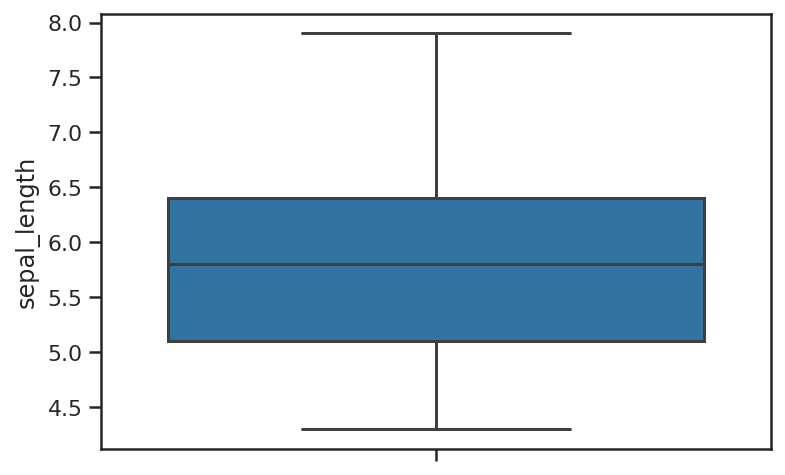``````sns.boxplot(y=df_iris["sepal_length"], orient='h')
``````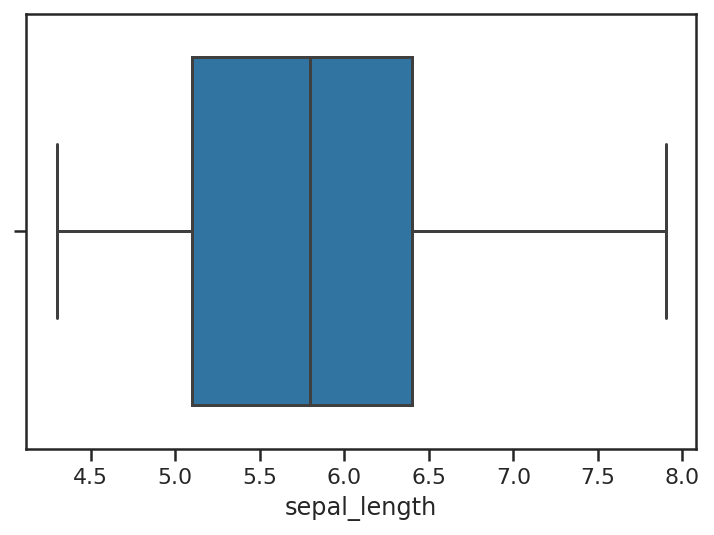### Grouped boxplot

It is grouped boxplots which are arguably most useful. These are also very easy to create in Seaborn. These require two columns from your Pandas dataframe. The `X` column contains the class for each of the rows of data (i.e. the species in the Iris dataset), while the `y` column contains the metric you wish to plot (i.e. `sepal_length`). You get back a boxplot for each class found, with the position of the plot on the axis denoting where the points lie.

``````sns.boxplot(x=df_iris["species"],
y=df_iris["sepal_length"])
``````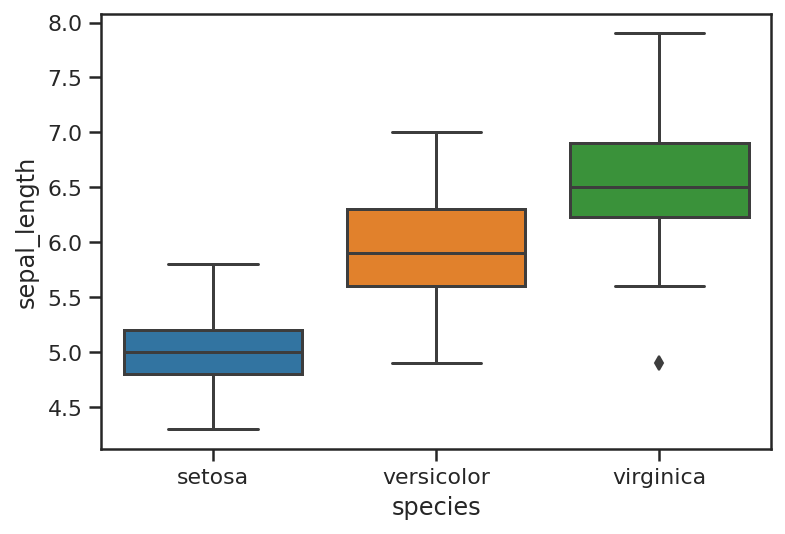### Boxplot theming

Seaborn provides a number of standard themes that you can apply to boxplots and any other Seaborn visualisations. To load these you simply call the `set_style()` function and pass in the name of the theme. Here are the main Seaborn themes.

#### Whitegrid theme

``````sns.set_style("whitegrid")
sns.boxplot(x=df_iris["species"],
y=df_iris["sepal_length"])
``````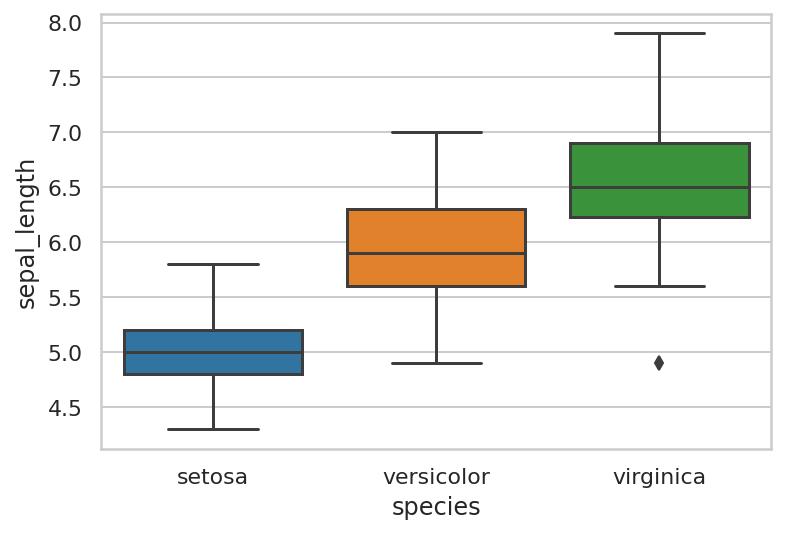#### Dark theme

``````sns.set_style("dark")
sns.boxplot(x=df_iris["species"],
y=df_iris["sepal_length"])
``````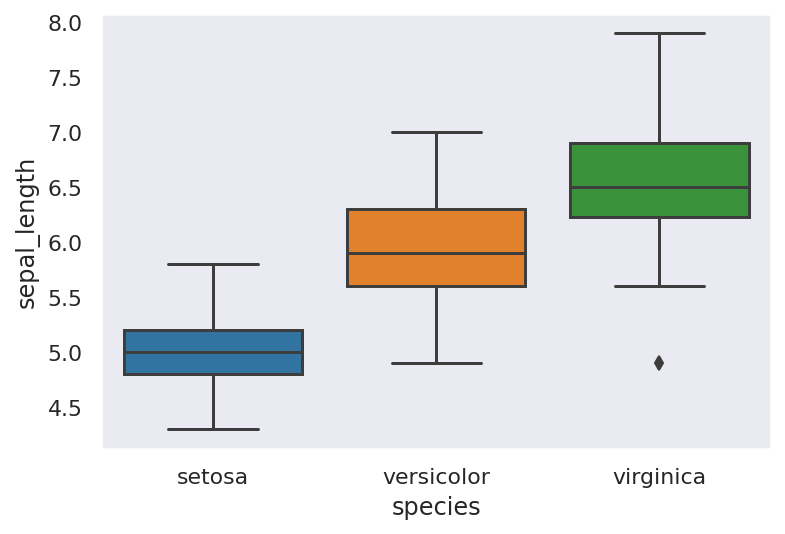#### White theme

``````sns.set_style("white")
sns.boxplot(x=df_iris["species"],
y=df_iris["sepal_length"])
``````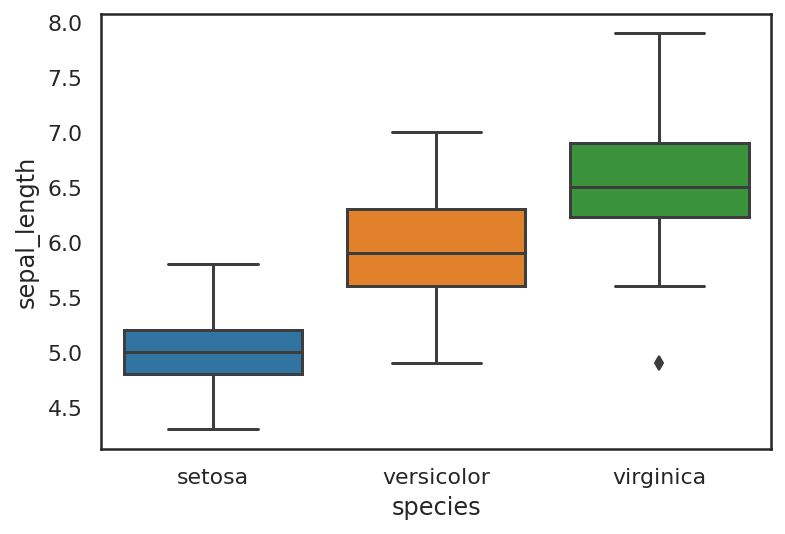#### Ticks theme

``````sns.set_style("ticks")
sns.boxplot(x=df_iris["species"],
y=df_iris["sepal_length"])
``````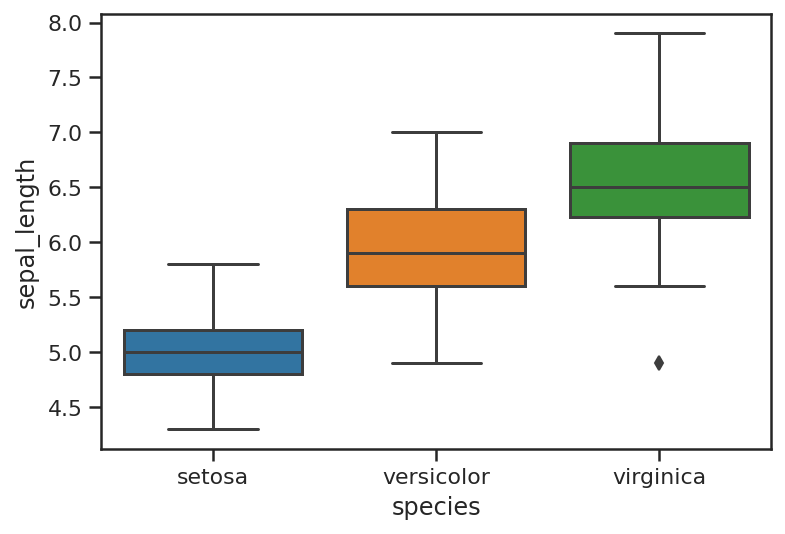### Boxplot colour palettes

Seaborn comes with loads of different standard colour palettes that you can load by passing in the palette name as an argument when calling the `boxplot()` function. Some of the main Seaborn colour palettes are shown below, but there are loads more on the Seaborn website.

#### Deep

``````sns.boxplot(x=df_iris["species"],
y=df_iris["sepal_length"],
palette="deep")
``````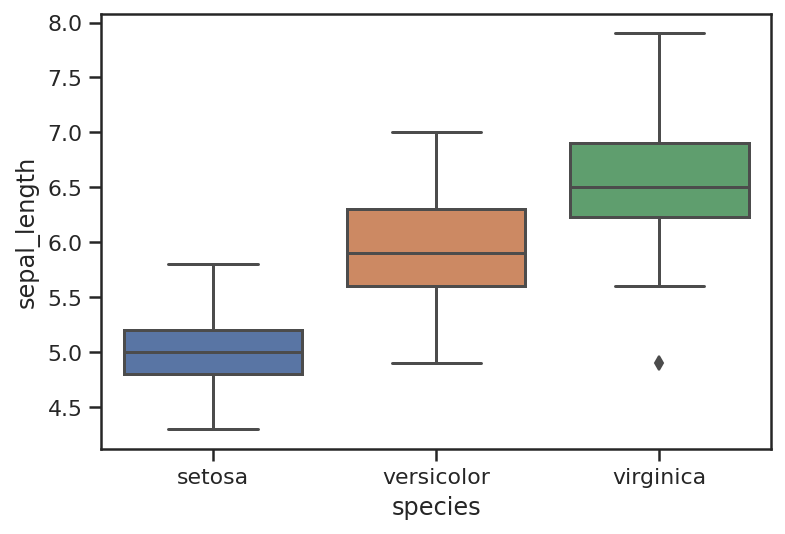#### Muted

``````sns.boxplot(x=df_iris["species"],
y=df_iris["sepal_length"],
palette="muted")
``````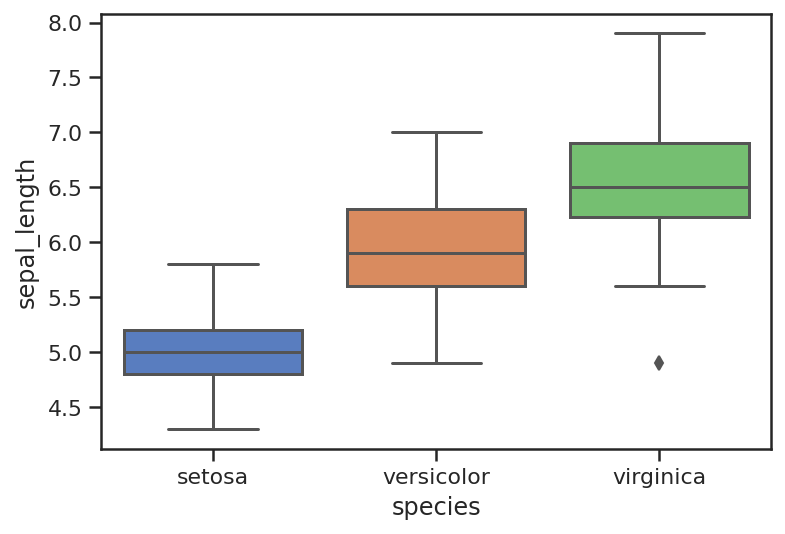#### Pastel

``````sns.boxplot(x=df_iris["species"],
y=df_iris["sepal_length"],
palette="pastel")
``````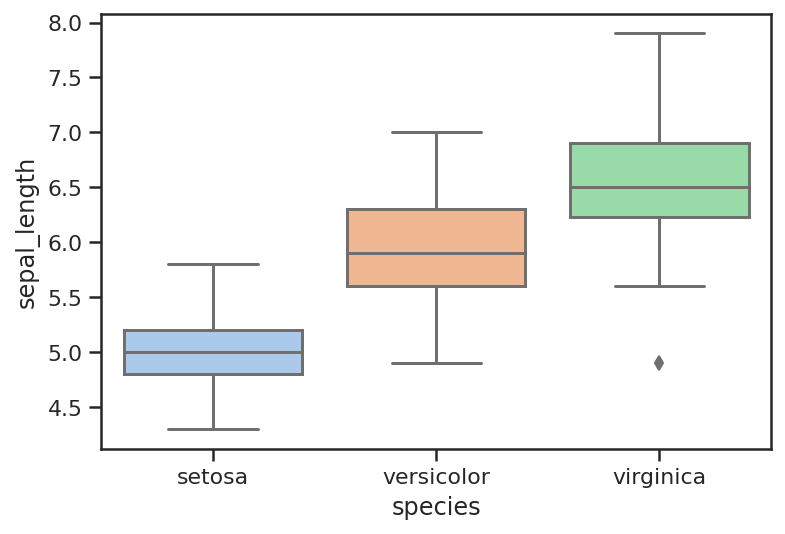#### Bright

``````sns.boxplot(x=df_iris["species"],
y=df_iris["sepal_length"],
palette="bright")
``````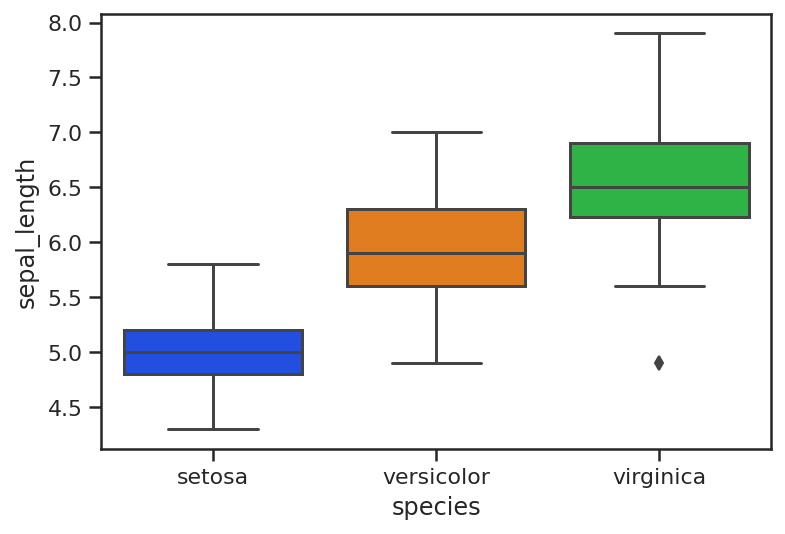#### Colorblind

``````sns.boxplot(x=df_iris["species"],
y=df_iris["sepal_length"],
palette="colorblind")
``````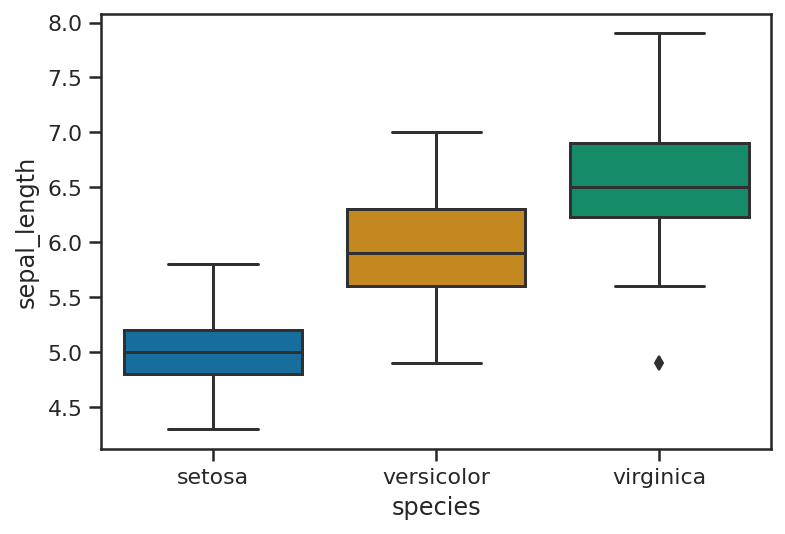#### Husl (HSLuv)

``````sns.boxplot(x=df_iris["species"],
y=df_iris["sepal_length"],
palette="husl")
``````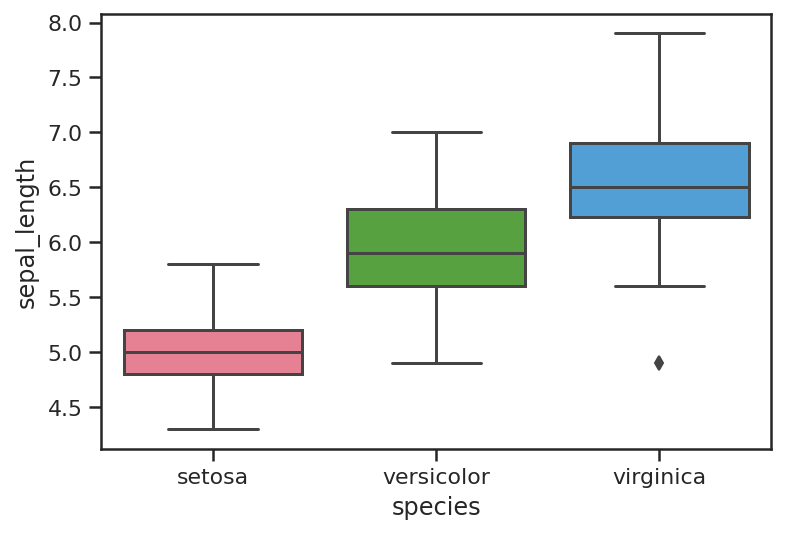### Boxplot styling

You can also control the width of the boxplots and the width of the line thickness, which can make them clearer to read on Powerpoint presentations. The thickness of the boxplot lines is controlled via the `linewidth` argument, while the boxplot width is controlled via `width`.

``````sns.boxplot(x=df_iris["species"],
y=df_iris["sepal_length"],
linewidth=5,
palette="husl")
``````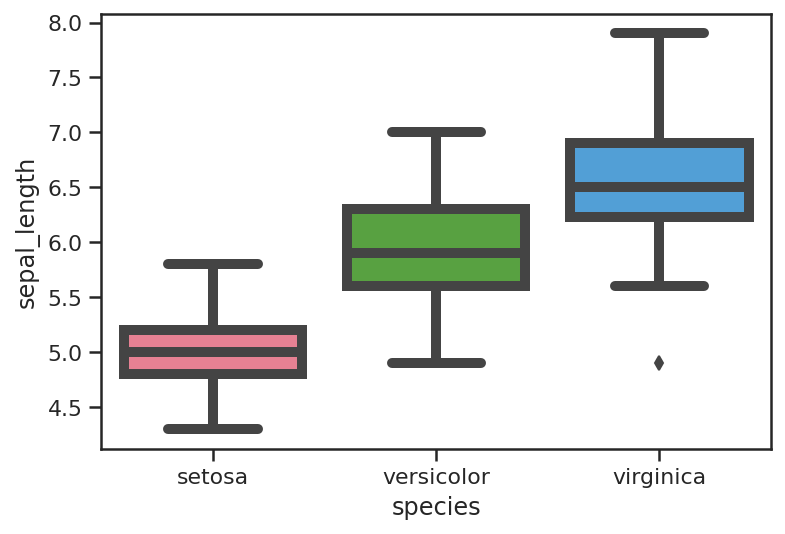``````sns.boxplot(x=df_iris["species"],
y=df_iris["sepal_length"],
width=0.3)
``````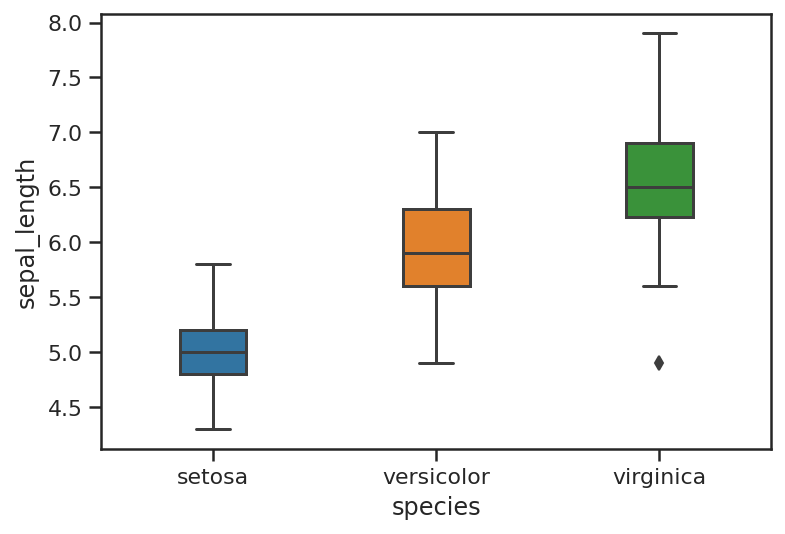### Viewing outliers with boxplots

If you have outliers in your data set, you’ll see a line of dots or diamonds above the top whisker. This alone is a very good reason to create boxplots when you’re building models, as it’s these outliers that can often throw a model off track.

``````sns.boxplot(x="day", y="total_bill", data=df_tips)
``````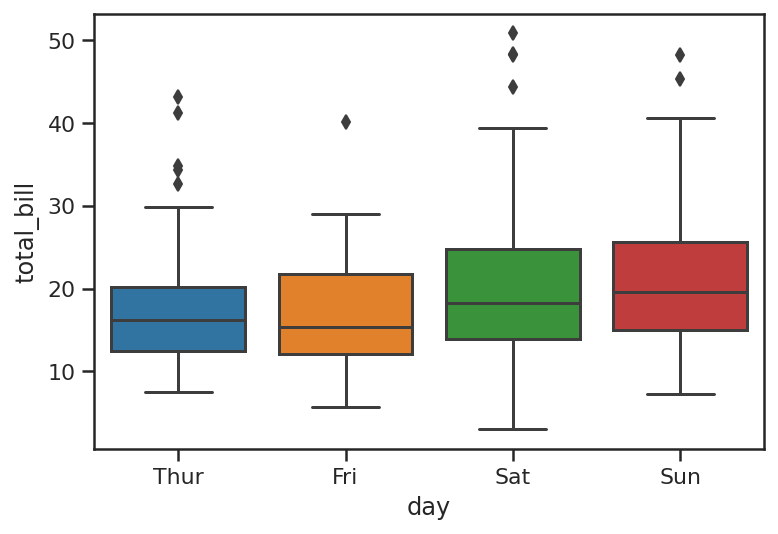The swarmplot or beeswarm plot is a related visualisation and can work well when plotted on top of a boxplot or violinplot. Cleverly, the points are aligned so they don’t overlap, but they only really work on datasets with smaller numbers of observations. Violinplots do a similar thing and scale better to larger volumes of data.

``````ax = sns.boxplot(x="day", y="total_bill", data=df_tips)
ax = sns.swarmplot(x="day", y="total_bill", data=df_tips, color=".25")
``````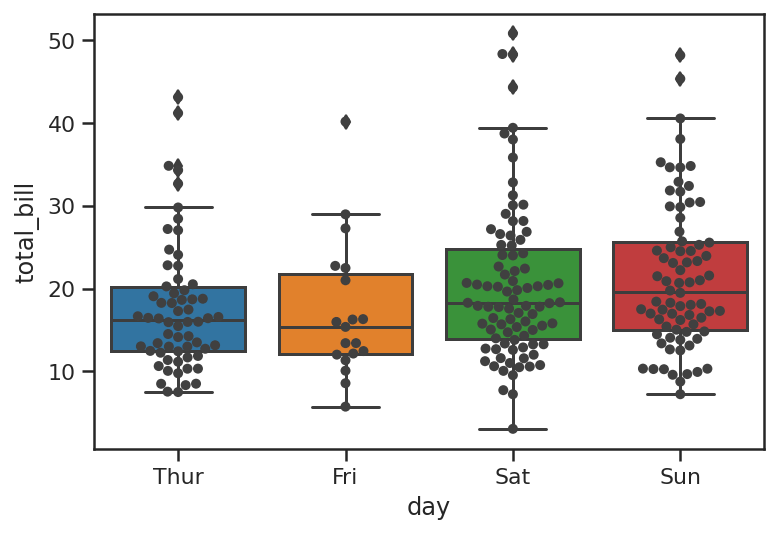Matt Clarke, Saturday, March 06, 2021

Matt Clarke Matt is an Ecommerce and Marketing Director who uses data science to help in his work. Matt has a Master's degree in Internet Retailing (plus two other Master's degrees in different fields) and specialises in the technical side of ecommerce and marketing.